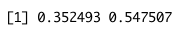# Calculate a Binomial Confidence Interval in R (3 Examples)

In this article you’ll learn how to calculate a binomial confidence interval in the R programming language.

We can calculate a binomial confidence interval using this formula:

$$p \pm z\times\sqrt{\frac{p\times(1-p)}{n}}$$

Being,

n: the sample size of our population
p: the proportion of cases of success
z: the z-value we choose

The table of content is structured as follows:

Let’s see how this can be done.

## Data Sample

For this tutorial, we will need to create some example data:

x <- 45 # Number of successes
n <- 100 # Sample size

As you can see above, we have defined two variables in order to calculate the confidence interval. Now, we are ready to see the three different examples we can use in order to calculate the confidence intervals.

## Example 1: Calculate Binomial Confidence Interval Using the binconf() Function

In order to use the binconf() function, please install the Hmisc package if you haven’t installed it yet. You just have to run the following command:

install.packages("Hmisc")

If you had already installed the “Hmisc” package before, you can skip the previous step. Just load the package before using the function:

library(Hmisc)
binconf(x, n,
alpha=.05)We have calculated the confidence interval with a confidence level of 95%, and that’s why alpha value equals to .05, but we can change this value depending on the confidence level we want to use.

Thus, the 95% confidence interval for the sample data we are using is [0.3561,0.5475].

## Example 2: Calculate Binomial Confidence Interval Using the prop.test() Function

Another example to calculate the 95% binomial confidence interval is using the prop.test() function.

prop.test(x,n,conf.level=.95,correct=FALSE)As shown, the confidence interval we obtain using the prop.test() function matches the one calculated with the previous method.

## Example 3: Calculate Binomial Confidence Interval Manually

We can also calculate the confidence interval manually. In order to accomplish this, first we need to define the proportion and the significance level.

p <- x / n # Calculate the proportion
a <- 0.05 # Define the significance level

Now, we define the function in order to calculate our interval. For this, we can use the qnorm and sqrt functions:

p +
c(-qnorm(1-a/2),qnorm(1-a/2)) *
sqrt((1/100)*p*(1-p))The output we obtain when we calculate the confidence interval manually is the same as when we calculate it using the binconf() or prop.test() functions.

## Video, Further Resources & Summary

Do you need more explanations on how to calculate confidence intervals in R? Then you should have a look at the following YouTube video of the Statistics Globe YouTube channel.

Furthermore, you could have a look at some of the other tutorials on Statistics Globe:

This post shows how to calculate a binomial confidence interval in R. In case you have further questions, you may leave a comment below.

Subscribe to the Statistics Globe Newsletter